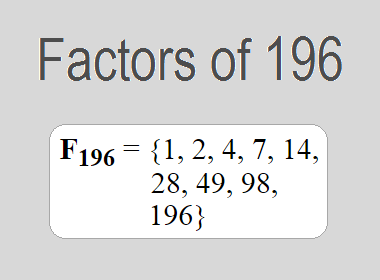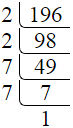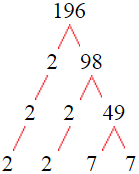# Factors of 196The factors of 196 are 1, 2, 4, 7, 14, 28, 49, 98, and 196 i.e. F196 = {1, 2, 4, 7, 14, 28, 49, 98, 196}. The factors of 196 are all the numbers that can divide 196 without leaving a remainder.

We can check if these numbers are factors of 196 by dividing 196 by each of them. If the result is a whole number, then the number is a factor of 196. Let's do this for each of the numbers listed above:

·        1 is a factor of 196 because 196 divided by 1 is 196.

·        2 is a factor of 196 because 196 divided by 2 is 98.

·        4 is a factor of 196 because 196 divided by 4 is 49.

·        7 is a factor of 196 because 196 divided by 7 is 28.

·        14 is a factor of 196 because 196 divided by 14 is 14.

·        28 is a factor of 196 because 196 divided by 28 is 7.

·        49 is a factor of 196 because 196 divided by 49 is 4.

·        98 is a factor of 196 because 196 divided by 98 is 2.

·        196 is a factor of 196 because 196 divided by 196 is 1.

## How to Find Factors of 196?

1 and the number itself are the factors of every number. So, 1 and 196 are two factors of 196. To find the other factors of 196, we can start by dividing 196 by the numbers between 1 and 196. If we divide 196 by 2, we get a remainder of 0. Therefore, 2 is a factor of 196. If we divide 196 by 3, we get a remainder of 1. Therefore, 3 is not a factor of 196.

Next, we can check if 4 is a factor of 196. If we divide 196 by 4, we get a remainder of 0. Therefore, 4 is also a factor of 196. We can continue this process for all the possible factors of 196.

Through this process, we can find that the factors of 196 are 1, 2, 4, 7, 14, 28, 49, 98, and 196. These are the only numbers that can divide 196 without leaving a remainder.

********************

********************

## Properties of the Factors of 196

The factors of 196 have some interesting properties. One of the properties is that the sum of the factors of 196 is equal to 399. We can see this by adding all the factors of 196 together:

1 + 2 + 4 + 7 + 14 + 28 + 49 + 98 + 196 = 399

Another property of the factors of 196 is that the prime factors of 196 are 2, and 7 only.

## Applications of the Factors of 196

The factors of 196 have several applications in mathematics. One of the applications is in finding the highest common factor (HCF) of two or more numbers. The HCF is the largest factor that two or more numbers have in common. For example, to find the HCF of 196 and 224, we need to find the factors of both numbers and identify the largest factor they have in common. The factors of 196 are 1, 2, 4, 7, 14, 28, 49, 98, and 196. The factors of 224 are 1, 2, 4, 7, 8, 14, 16, 28, 32, 56, 112, and 224. The largest factor that they have in common is 28. Therefore, the HCF of 196 and 224 is 28.

Another application of the factors of 196 is in prime factorization. Prime factorization is the process of expressing a number as the product of its prime factors. The prime factors of 196 are 2, and 7, since these are the only prime numbers that can divide 196 without leaving a remainder. Therefore, we can express 196 as:

196 = 2 × 2 × 7 × 7

We can do prime factorization by division and factor tree method also. Here is the prime factorization of 196 by division method,196 = 2 × 2 × 7 × 7

Here is the prime factorization of 196 by the factor tree method,196 = 2 × 2 × 7 × 7

## Conclusion

The factors of 196 are the numbers that can divide 196 without leaving a remainder. The factors of 196 are 1, 2, 4, 7, 14, 28, 49, 98, and 196. The factors of 196 have some interesting properties, such as having a sum of 399. The factors of 196 have several applications in mathematics, such as finding the highest common factor and prime factorization.